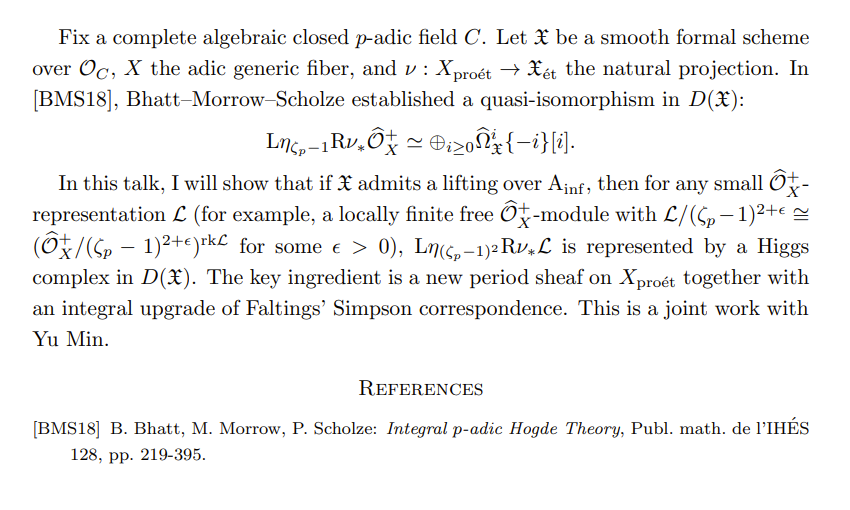# PKU/BICMR Number Theory Seminar - 2023

## Venue

• Until further notice, the offline or hybrid talks will be held in room 77201, BICMR.
• For online or hybrid talks, the Zoom number is 743 736 2326, and the password is 013049.

## Schedule

The series is also announced on researchseminars.org

 Time and Date Speaker Topic Slides / Recording March 15 15:00-16:00 Yupeng Wang (Chinese Academy of Sciences) Integral $$p$$-adic non-abelian Hodge theory for small representationsVideo April 5 15:30-16:30 Li Lai (Tshinghua University) On the irrationality of certain $$2$$-adic zeta values Let $$\zeta_2(\cdot)$$ be the Kubota-Leopoldt $$2$$-adic zeta function. We prove that, for every nonnegative integer $$s$$, there exists an odd integer $$j$$ in the interval $$[s+3,3s+5]$$ such that $$\zeta_2(j)$$ is irrational. In particular, at least one of $$\zeta_2(7),\zeta_2(9),\zeta_2(11),\zeta_2(13)$$ is irrational. Our approach is inspired by the recent work of Sprang. We construct explicit rational functions. The Volkenborn integrals of the (higher order) derivatives of these rational functions produce good linear combinations of $$1$$ and $$2$$-adic Hurwitz zeta values. The most difficult step is to prove that certain Volkenborn integrals are nonzero, which is resolved by careful manipulation of the binomial coefficients. Slides Video April 12 15:30-16:30 Fan Gao (Zhejiang University) Some satisfactory and unsatisfactory aspects of the dual groups for central covers We consider finite degree central covers of a linear reductive group in the local setting. Using some examples as the highlights, we will explain the dual group of such a central cover, and illustrate how much it captures the representation-theoretic information of the central cover, and also how much it fails for the same purpose. We concentrate on two aspects of a representation: formal degree and wavefront set. Video April 26 15:30-16:30 King Fai Lai 同调代数的一个注记 谈一谈关于非交换环的同调代数的几方面。 Slides May 17 15:00-16:00 Juan Esteban Rodríguez Camargo (MPIM) Solid locally analytic representations (Joint with Joaquín Rodrigues Jacinto) In this talk I will introduce different categories of $$p$$-adic representations in the framework of condensed mathematics. We give different geometric interpretations to them, construct explicit adjunctions that serve to compare cohomology theories, and see an application to $$p$$-adic categorical local Langlands for $$\mathrm{GL}_1$$. Video May 31 15:30-16:30 Koji Shimizu (YMSC) Robba site and Robba cohomology We will discuss a $$p$$-adic cohomology theory for rigid analytic varieties with overconvergent structure (dagger spaces) over a local field of characteristic $$p$$. After explaining the motivation, we will define a site (Robba site) and discuss its basic properties. June 7 09:00-10:00 Alexander Bett (Harvard University) p-adic obstructions and Selmer sections In 1983, shortly after Faltings' resolution of the Mordell Conjecture, Grothendieck formulated his famous Section Conjecture, positing that the set of rational points on a projective curve Y of genus at least two should be equal to a certain section set defined in terms of the etale fundamental group of Y. To this day, this conjecture remains wide open, with only a small handful of very special examples known. In this talk, I will discuss recent work with Jakob Stix, in which we proved a Mordell-like finiteness theorem for the "Selmer" part of the section set for any smooth projective curve Y of genus at least 2 over the rationals. This generalises the Faltings-Mordell Theorem, and implies strong constraints on the finite descent locus from obstruction theory. The key new idea in our proof is an adaptation of the recent proof of Mordell by Lawrence and Venkatesh to the study of the Selmer section set. Time permitting, I will also briefly describe recent work with Theresa Kumpitsch and Martin Lüdtke in which we compute the Selmer section set in one example using the Chabauty-Kim method. Video June 21 14:30-15:30 Rui Chen (Zhejiang University) Ext-vanishing result for Gan-Gross-Prasad model In this talk we will show that the Ext-analogue of GGP model vanishes for tempered representations, as conjectured by D. Prasad. As a corollary, this implies that the geometric multiplicity equals the Euler-Poincare characteristic. Slides July 19 09:00-10:00 Lie Qian (Stanford University) Local Compatibility for Trianguline Representations Trianguline representations are a big class of $$p$$-adic representations that contain all nice enough (cristalline) ones but allow a continuous variation of weights. Global consideration suggests that the $$GL_2(\mathbb{Q}_p)$$ representation arising from a trianguline representation should have nonzero eigenspace under Emerton's Jacquet functor. We prove this result using purely local method as well as a generalization to $$p$$-adic representation of $$G_F$$ for $$F$$ unramified over $$\mathbb{Q}_p$$. September 4 15:00-16:00 Jiajun Ma (Xiamen University) Applications of Hecke Algebra in the Representation of Reductive Groups Consider a reductive linear algebraic group G. Let  H be the generic Hecke algebra attached to the Weyl group of G. The representations of G and H have many deep connections. In this talk, I will discuss our two recent works where Hecke algebras play a crucial role:   1. Counting special unipotent representations of real reductive groups  2. Determining the theta correspondence over finite fields.I will also discuss the analog picture in the theta correspondence over p-adic fields when time permits. Slides September 13 14:00-15:00 Chenji Fu (University of Bonn) Explicit categorical mod $$\ell$$ local Langlands correspondence for depth-zero supercuspidal part of $$\mathrm{GL}_2$$ Let F be a non-archimedean local field. I will explicitly describe: (1) (The category of quasicoherent sheaves on) The connected component of the moduli space of Langlands parameters over $$\overline{\mathbb{Z}_l}$$ containing an irreducible tame L-parameter with $$\overline{\mathbb{F}_l}$$ coefficients; (2) the block of the category of smooth representations of $$G(F)$$ with $$\overline{\mathbb{Z}_l}$$ coefficients containing a depth-zero supercuspidal representation with $$\overline{\mathbb{F}_l}$$ coefficients. The argument works at least for (simply connected) split reductive group $$G$$, but I will focus on the example of $$\mathrm{GL}_2$$ for simplicity. The two sides turn out to match abstractly. If time permits, I will explain how to get the categorical local Langlands correspondence for depth-zero supercuspidal part of $$\mathrm{GL}_2$$ with $$\overline{\mathbb{Z}_l}$$ coefficients in Fargues-Scholze's form. September 13 15:30-16:30 Chunyi Li (University of Warwick) Bridgeland 稳定性条件简介（代数几何角度） 三角范畴上的稳定性条件是本世纪初 Bridgeland 受到 Douglas 在理论物理学中相应研究的启发给出的一个概念。由于其数学定义严谨，简明，且适用性较为广泛，在过去的二十年中相关交叉学科都有对其消化和应用。Bridgeland 稳定性条件也从而得到了快速和较为深入的研究。本报告将从代数几何角度背景出发简单介绍相关的概念和关于射影复流形的稳定性条件空间目前一些已知的结论。